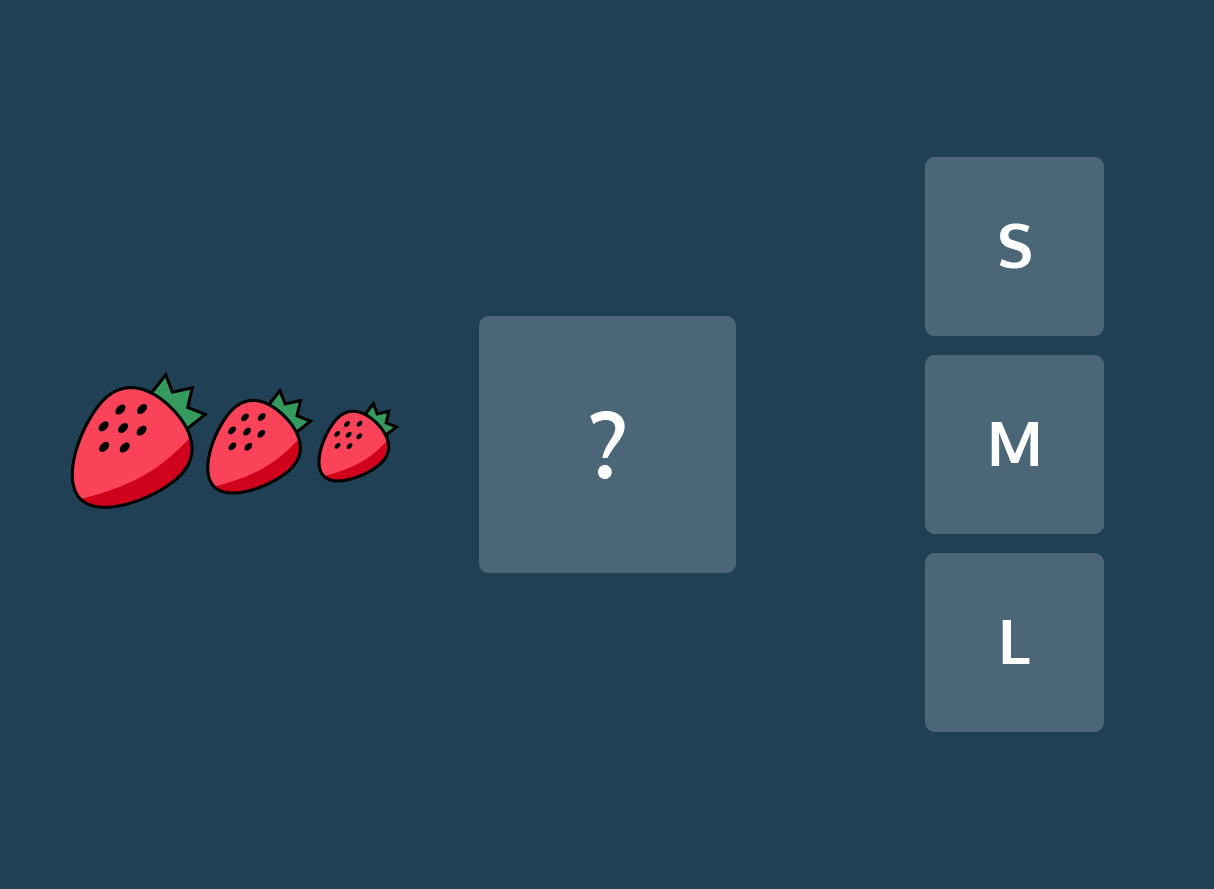# Regression vs. Classification

Learn about the two types of Supervised Learning algorithms.

Machine Learning is a set of many different techniques that are each suited to answering different types of questions.

We have previously divided algorithms into two groups — Supervised Learning vs Unsupervised Learning. Supervised learning algorithms use labeled data as input while unsupervised learning algorithms use unlabeled data. However, we can further distinguish machine learning algorithms by the output they produce. In terms of output, two main types of machine learning models exist: those for regression and those for classification.

### Regression

Regression is used to predict outputs that are continuous. The outputs are quantities that can be flexibly determined based on the inputs of the model rather than being confined to a set of possible labels.

For example:

• Predict the height of a potted plant from the amount of rainfall
• Predict salary based on someone’s age and availability of high-speed internet
• Predict a car’s MPG (miles per gallon) based on size and model yearLinear regression is the most popular regression algorithm. It is often underrated because of its relative simplicity. In a business setting, it could be used to predict the likelihood that a customer will churn or the revenue a customer will generate. More complex models may fit this data better, at the cost of losing simplicity.

### Classification

Classification is used to predict a discrete label. The outputs fall under a finite set of possible outcomes. Many situations have only two possible outcomes. This is called binary classification (True/False, 0 or 1,/ not).

For example:

• Predict whether an email is spam or not
• Predict whether it will rain or not
• Predict whether a user is a power user or a casual user

Multi-label classification is when there are multiple possible outcomes. It is useful for customer segmentation, image categorization, and sentiment analysis for understanding text. To perform these classifications, we use models like Naive Bayes, K-Nearest Neighbors, and SVMs.Choosing a model is a critical step in the Machine Learning process. It is important that the model fits the question at hand. When you choose the right model, you are already one step closer to getting meaningful and interesting results.